# Shedding Light on Acids and Bases Episode 5: Advanced Acid-Base Chemistry

In Episode 5, Advanced Acid-Base Chemistry, we take a deeper look at pH and explain how the numbers on the pH scale are actually related to the number of H+ or OH- ions present in an acid or a base. We then descend even deeper down—to the atomic level—to explain what actually happens when acids react with bases and what actually happens when acids react with metals. We introduce students to ionic equations and to the role (or lack thereof) of spectator ions in many chemical reactions. This episode can be thought of as an extension to middle school acid-base chemistry or as an introduction to senior school acid-base chemistry.
A 5½-minute excerpt followed by a 1½-minute trailer.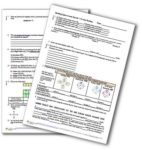The Episode 5 Question Sheet for Students:The PDF version.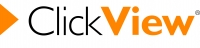If you have ClickView, watch the whole episode here.

Coming Soon To…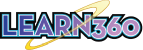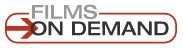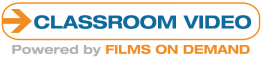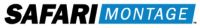Don’t have any of the above? Rent or buy the Shedding Light series and/or individual programs from our Vimeo page!!

The Transcript (which can be used as a textbook)

Contents
Part A: Intro and Recap
Part B: H+ ions and pH
Part C: H+ Numbers Count
Part D: Opposites Attract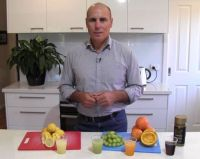Part A: Intro and Recap.

Hi everyone. Welcome to the fifth episode of the Shedding Light on Acids and Bases series. In this episode, we’re going to look at some more advanced concepts that relate to the chemistry of acids and bases.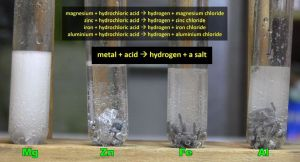We’re first going to quickly go over some of the basics that we’ve already looked at in Episodes 1 to 4 but then get into more detail of how and why metals react with acids and how and why neutralization reactions occur. We’re also going to look at the pH scale in more detail. Why were the numbers 0-14 chosen and what do the numbers actually mean?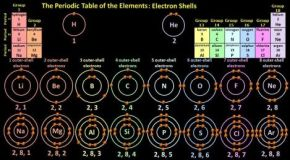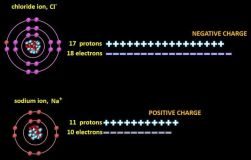It will certainly be beneficial if you’re familiar with electron shells, and both covalent and ionic bonding before watching this video. These topics are covered in the Shedding Light on Atoms series, but I will cover the basics anyway as I go through the more advanced topics relating to acids and bases.

So what do we already know?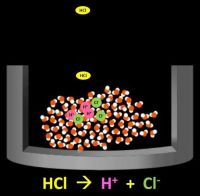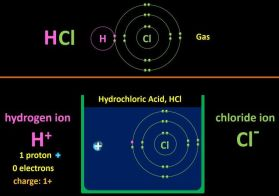All acids produce H+ ions when they dissolve in water. Hydrochloric acid for example has the chemical formula HCl. Pure HCl is a gas, but when it dissolves in water, a H+ ion and a Cl ion are formed. A H+ ion is a hydrogen atom without an electron, so it is in fact just a single positively charged proton. Acids are fairly reactive because the H+ ions have a fairly strong attraction to the negatively charged electrons of other atoms. H+ ions typically join onto water molecules to form hydronium ions, which have the formula H3O+.

Acids are not really liquids. They’re solutions. Solutions are basically mixtures where a chemical, like HCl, is dissolved in a liquid. In acids, that liquid is always water.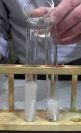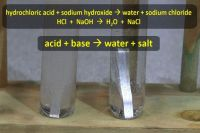When an acid reacts with a metal, hydrogen gas and a salt are produced.

These two test tubes contain magnesium and hydrochloric acid and you can see the hydrogen gas being produced. Now when water is added to the test tube on the left, the acid gets watered down but the reaction continues. When a base (in this case sodium hydroxide) is added to the test tube on the right, the acid and the base neutralize each other and the magnesium stops reacting with the acid because all the acid reacts with the base and there’s literally no acid left in the test tube. So, when an acid reacts with a base, salt and water are produced.

When an acid reacts with a carbonate (which is an example of a base), a salt, water, and carbon dioxide are produced.

Bases are chemicals that react with acids to produce water and a salt. When bases dissolve in water, they produce hydroxide ions. (OH)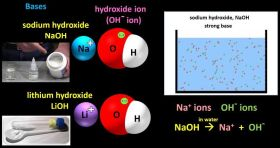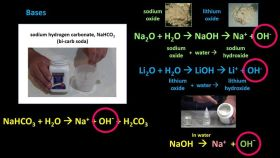The most straight forward examples are compounds like sodium hydroxide and lithium hydroxide which are made in part of hydroxide ions. When they dissolve in water, they split apart and you get OH ions floating around the water. The OH ions can then readily react with acids and with other chemicals.

Some carbonates like sodium hydrogen carbonate (bi-carb soda) are water soluble and these too produce OH ions when they dissolve in water, so carbonates are bases as well.

In a similar way, some oxides, like sodium oxide and lithium oxide also react with water and produce OH ions. So bases generally produce OH ions when they dissolve in water. OH ions, OH ions, OH ions.

Acids and bases can be strong or weak depending on how many H+ ions or OH ions they produce in water.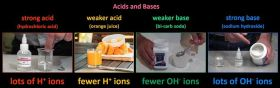Strong acids, like hydrochloric acid, contain lots of H+ ions, while weaker acids like the juice of an orange contain fewer H+ ions. Strong bases, like sodium hydroxide, contain lots of OH ions, and weaker bases, like bi-carb soda, contain fewer OH ions.

Pure water is neutral. It is neither an acid nor a base.

The pH scale is the scale used to express just how acidic or basic a solution is. It goes from 0 to 14.

Pure water has a pH of 7. Anything with a pH of less than 7 is acidic and the further from 7 it is the more and more strongly acidic it is. By more strongly acidic, I mean that there are more H+ ions in a given volume. A cup of lemon juice, for example, has more H+ ions than a cup of orange juice. Anything with a pH of more than 7 is basic and the further from 7 it is, the more and more strongly basic it is, that is, there are more and more OHions present.

So that what a very quick summary of what we had already covered in the first four episodes of the series. So let’s get into some detail starting with the concept of pH. What exactly is pH? How many H+ ions do you need to have a solution with a pH of 4 or 1 whatever? Let’s take a look.

Part B: H+ ions and pH.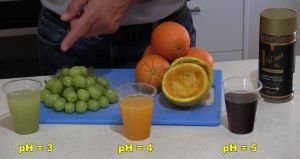The pH scale is what’s called a logarithmic scale. Let me explain.

A typical coffee has a pH of about 5, so it’s a very weak acid, orange juice has a pH of about 4, so it’s a stronger acid, and grapes have a pH of about 3, again stronger. Now for every change of 1 on the logarithmic pH scale there’s a 10-fold change in the number of H+ ions, assuming you’ve got the same amount of liquid of course.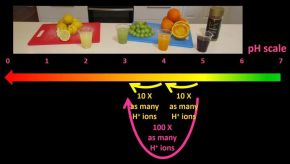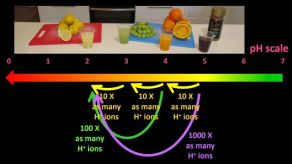So a cup of orange juice has 10 times as many H+ ions as a cup of coffee does, and a cup of grape juice has 10 times as many H+ ions as a cup of orange juice. So, mathematically it makes sense that a cup of grape juice have 100 times as many H+ ions as a cup of coffee does. Lemon juice, with a pH of 2, has 10 times as many H+ ions as a cup of grape juice, 100 times as many H+ ions as a cup of orange juice, and 1000 times as many H+ ions as a cup of coffee. That’s how the pH scale works.

It’s the same on the base side of the pH scale.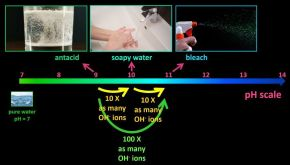Soapy water, with a pH of 10, has 10 times the concentration of OH ions as an antacid mixture of pH 9, and bleach, with a pH of 11, has ten times the concentration of OH ions as a soapy water. So, bleach has 100 times as many OH ions as an equal amount of antacid mixture.

So, a logarithmic scale is a scale where each number represents 10 times more than the previous number. Logarithmic scales make it easier to express huge variations in quantities.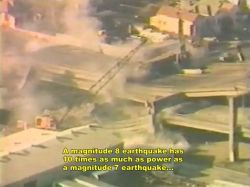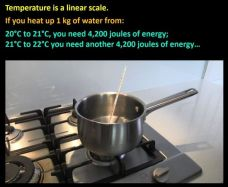The power of earthquakes is also measured on a logarithmic scale. A magnitude 8 earthquake has 10 times as much as power as a magnitude 7 earthquake, which has ten times as much power as a magnitude 6 earthquake. A logarithmic scale is used because the strongest earthquakes have billions of times more energy than the weakest ones.

In contrast linear scales are probably what we’re more used to. Temperature is a linear scale. If you heat up 1 kg of water from 20°C to 21°C you need 4,200 joules of energy. To then go from 21°C to 22°C you need another 4,200 joules of energy and so on. Equal amounts of energy input create equal changes in temperature.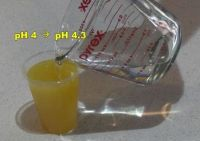Now, back to pH, this is half a cup of orange juice and it has a pH of 4. If I now water it down by half, the pH increases to only 4.3. You can halve the concentration and the pH doesn’t change that much!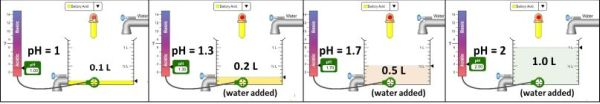Let me demonstrate with this simulation. I’ll first place this pH meter into this beaker.  If I pour 0.1 L of battery acid, that is, sulfuric acid, into the beaker we can see that it has a pH of 1, in other words, battery acid is very strongly acidic. If I now water down the acid with 0.1 L of water, so that the concentration is halved, the pH rises to only 1.3. If I now add more water and get to 0.5 L in the beaker, the acid concentration is now only 1/5 of what it was originally (there are now 4 parts water and 1 part battery acid), but the pH is still only 1.7; it hasn’t changed all that much. If I now add another half a litre of water so that the acid’s concentration gets to only 1/10 of what it was originally, we can see that the pH rises to 2.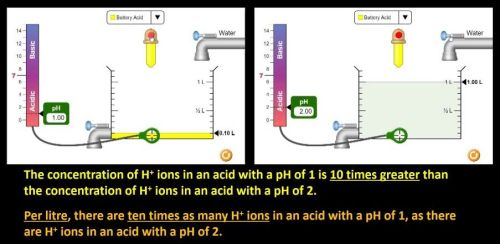So, let me say again, the concentration of H+ ions in an acid with a pH of 1 is 10 times greater the concentration of H+ ions in an acid with a pH of 2. I can also say that per litre, there are ten times as many H+ ions in an acid with a pH of 1, as there are H+ ions in an acid with a pH of 2.

An increase in pH from 1 to 2 means the concentration of H+ ions decreases by a factor of 10.

I can now drain my pH 2 acid, leaving just 0.1 litres of it and then add water again so that the concentration of H+ ions again decreases by a factor of 10. The pH before I added water was 2, and since its concentration is now only 1/10 of what it was, its pH is now 3. Again a 10-fold decrease in H+ concentration causes a change in pH of 1, from 2 to 3 this time.

If I now drain the heavily watered-down battery acid again and then water it down again by a factor of 10, the pH rises to 4.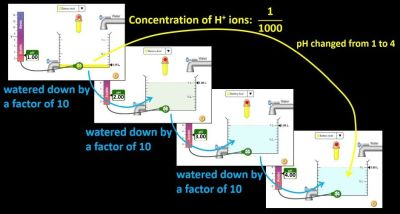So, I started with an acid with a pH of 1, it was watered down by a factor of 10, and then watered down by a factor of 10 again and then watered down by a factor of 10 again, so the concentration of H+ ions in the final watered-down solution was 1/1000 of the original concentration and the pH changed from 1 to 4.

Of course the reverse is also true. If the concentration of H+ ions increases by a factor of 10, the pH will change by 1 the opposite way.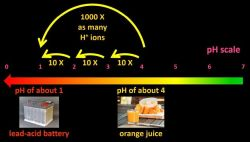So if we look at just orange juice and the acid in car batteries, which are actually called lead-acid batteries, with pH’s of about 4 and 1 respectively, then 1 litre of battery acid has 10 x 10 x 10, that is, 1000 times as many H+ ions as a litre of orange juice.

If orange juice gets in your eyes it stings a little but we can obviously drink orange juice.

Sulfuric acid though, won’t just sting if gets in your eyes, let me put it that way, and you definitely don’t want to drink it.

As I said, logarithmic scales allow you to express huge changes in the amounts of something using only relatively small numbers. But how many H+ ions are actually in a litre of orange juice or a cup of coffee or whatever? Let’s take a look at the actual numbers.

Part C: H+ Numbers Count

The pH of an acid is actually determined by how many H+ ions there are per litre of the acid.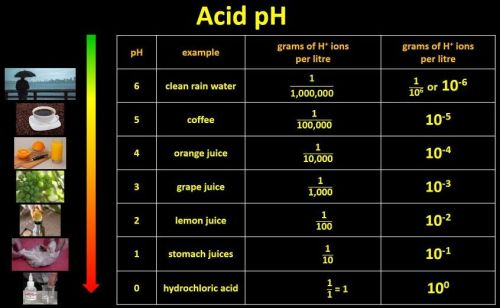This table will lay out the numbers. If a weak acid like clean rainwater has a pH of 6, it turns out that there is 1/1,000,000 th of a gram of H+ ions in each litre of the weak acid. Notice that there are 6 zeros in 1,000,000! 6 zeros, pH 6. An acid with a pH of 5, like coffee (which is more acidic), contains 1/100,000th of a gram of H+ ions per litre of the acid. Notice that there are 5 zeros in 100,000 (and that there are 10 times as many H+ ions as the previous row). This simple trend continues. pH 4 acids have 1/10,000 th of a gram of H+ ions (notice 4 zeros) per litre, and so on (let me fill in the table) down to pH 0 acids, which have 1 1nth of a gram (zero zeros) which is just 1 of course. Acids with a pH of 0 contain about 1 gram of H+ ions per litre.

Now 1,000,000 can also be expressed as 106, so 1/1,000,000th can also be written as 1/106 grams of H+ ions per litre, or 10-6. If you have this many H+ ions per litre, the pH is 6. 10-5 grams of H+ ions per litre produce a pH of 5 and so on. So, pH is not some random scale, it’s based on the amount of H+ ions per litre of the acid. The scale was originally devised by Danish scientist Søren Peder Lauritz Sørensen in 1909.

The H stands for hydrogen, but the funny thing is, no-one knows exactly why he used the “p” or what it stands for. It may be p for “power” of hydrogen, or p for “potential” of hydrogen. No-one knows. I can’t believe that no-one asked him at the time! But everyone liked his idea and went with it.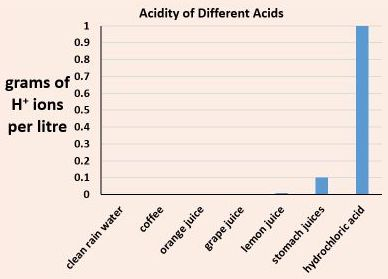The huge differences in the number of H+ ions in different acids makes a logarithmic scale so much easier to use. If you had to graph the number of H+ ions in different acids, it would be impossible using a linear scale, which here goes up 0.1, 0.2, 0.3 and so on, because only the very strongest acids show up. Theses ones here are so close to zero that you can’t even them. Also the numbers are pretty difficult to get your head around. 0.001, (10-3) for example, might be reasonably okay, but an acid with a pH of 3.5 contains 0.00032 g of H+ ions per litre and an acid with a pH of 2.2 contain 0.0063 grams of H+ ions per litre. These numbers are even more difficult to get your head around. Using pH is much easier. However, in senior chemistry you may actually have to calculate the amount of H+ ions in a given volume of an acid but your teachers will teach you all the formulas that you need of course when you come to it.

So what does a pH of 7 mean? Why does pure water, which is neutral have a pH of 7?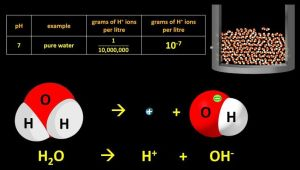Well, if we follow the pattern in the table, a litre of pure water, pH of 7, has 1/10,000,000 of a gram of H+ ions floating around in it. Notice that there are 7 zeros. Where do the H+ ions come from? Well, we know the formula for water is H2O. It turns out that just completely randomly as they bounce around each other, a tiny tiny tiny number of H2O molecules just split apart and form H+ ions and OH ions. They bounce around for a little while and then join up again (not usually with their original partners) and this just happens constantly. So, at all times, pure water has a tiny number of H+ ions floating around in it and an equally tiny number of OH ions floating around in it. It’s neither acidic nor basic; it’s neutral.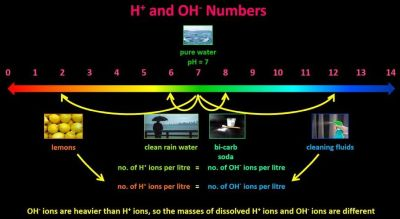So what does a pH of above 7 mean?

Well, the number of H+ ions per litre in an acid with a pH of 6 equals the number of OH ions per litre in a base with a pH of 8. Both of these numbers are the same distance from 7 of course, 7 being neutral.

Likewise the number of H+ ions per litre in an acid with a pH of 2 equals the number of OH ions per litre in a base with a pH of 12. Both of these pH’s are 5 away from the neutral 7. It’s a pretty simple concept really. However, while the numbers are the same, OH ions are heavier than H+ ions, so the masses of dissolved H+ ions and OH ions are different.

So, what happens when an acid and a base are mixed? Well, we know that a neutralization reaction occurs and a salt and water are formed. But, this is a little misleading. Let’s take a deeper look.

Part D: Opposites Attract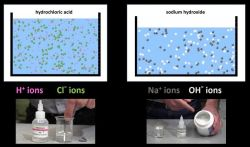The reason that acids are so reactive is that they produce H+ ions. H+ ions have a concentrated positive charge, and so they are strongly attracted to anything that has a negative charge. They don’t react with everything of course, but they are generally speaking quite reactive.

We know that hydrochloric acid is made of H+ ions and Cl ions floating around in water, and a solution of sodium hydroxide is made of Na+ and OH ions floating around in water.

When they’re both mixed in the test tube on the right, which initially contains magnesium and hydrochloric acid, neutralization occurs and we can tell because the Mg stops reacting. The equation: HCl + NaOH —> NaCl + H2O is accurate but it’s also a little misleading.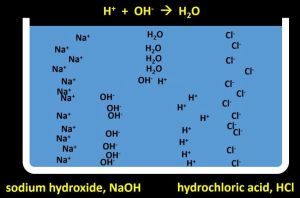If we start with HCl and NaOH in separate sections of a container and then remove the barrier allowing them to mix, the random movement of the H+ ions and the OH ions, which I’m simplifying enormously here of course, results in collisions between the two and they join together to form water! The equation can be written as H+ + OH —> H2O!!! The Na+ ions and the Cl ions stay dissolved in the water and just keep floating around, although they mix together of course not like I’m showing here. They don’t actually do anything so they’re called “spectator ions”, kind of as if they’re just watching on as the H+ ions join up with the OH ions. However, if all the water evaporated, the Na+ ions and the Cl ions would join up and form solid NaCl, table salt. This equation is called an ionic equation.

So, this is the basic equation for the reaction between hydrochloric acid and sodium hydroxide:

HCl + NaOH —> NaCl + H2O

However, these three things are dissolved in water, and this is water (liquid water), so to express that, scientists often use these symbols written as subscripts.

HCl(aq) + NaOH(aq) —> NaCl(aq) + H2O(l)

The “aq” stands for “aqueous”, which means dissolved in water. It’s related to the word aqua meaning water, so the equation shows that these three things are dissolved in water. The “l” stands for liquid.

Now since these three things all separate into ions in water, we can also write the equation like this:

H+(aq) + Cl(aq) + Na+(aq) + OH(aq) —> Na+(aq) + Cl(aq) + H2O(l)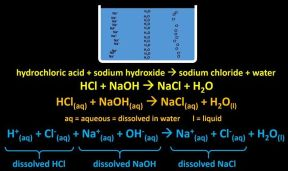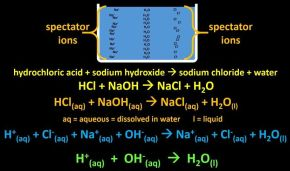These ions are the dissolved HCl, these the dissolved NaOH, and these the dissolved NaCl salt that is produced.

Now since the Na+ and the Cl ions appear on both sides of the equation, and they haven’t actually done anything, hence the name spectator ions, the equation simplifies down to this: H+(aq) + OH(aq) —> H2O(l). So writing NaCl(aq) is just a simple way of expressing the fact that we now have Na+ ions and Cl ions floating around in the water).

So all of these equations express what’s going on in a slightly different way and you’re likely to see more ionic equations as you continue your chemistry studies because ions are everywhere.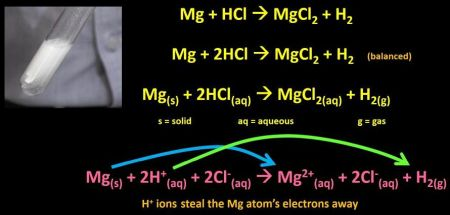Ions are also involved in the reaction between acids and metals. Here we can see magnesium metal chemically reacting with hydrochloric acid.

The simple equation is Mg + HCl —> MgCl2 + H2.

If we balance the equation we get Mg + 2HCl —> MgCl2 + H2

To this equation we can add the state that they’re in: Mg(s) + 2HCl(aq) —> MgCl2(aq) + H2(g).

The s stands for solid and the g stands for gas. The aq stands for, as we’ve seen, aqueous. So we can re-write the equation as an ionic equation: Mg(s) + 2H+(aq) + 2Cl(aq) —> Mg2+(aq) + 2Cl(aq) + H2(g).

When the H+ ions crash into the Mg atoms, they steal the Mg atom’s electrons away, so the Mg atoms become positively charged Mg2+ ions and the H+ ions become H atoms.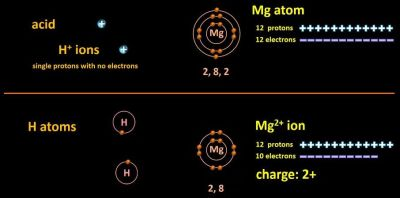Now why Mg2+? Let’s take a look at Mg’s electron shells.

An Mg atom has 12 protons in its nucleus (which I’m not showing) and 12 electrons. It has 2 electrons in its first shell, 8 in its second and 2 in its third, which is its outer shell. However, this situation doesn’t last long when magnesium is immersed in acid which contains H+ ions, basically single protons with no electrons floating around in the water. None of this is drawn to scale by the way. Let me double up so that we can get a before and after. When H+ ions approach an Mg atom, they each pull on one of the outer-shell electrons, leaving it two electrons short. The H+ ions become H atoms.

The magnesium atom becomes a magnesium ion and since it now has two more protons than electrons, 12 vs 10, it is said to have a charge of 2+. Its symbol is Mg2+. Now that it has 8 electrons in its outer shell, it’s quite stable, and it doesn’t react with any more H+ ions. Atoms tend to react until they end up with 8 electrons in their outer shells. The Mg2+ ion gets carried away into the water so the strip of magnesium you start with eventually gets eaten away. The two hydrogen atoms form a covalent bond and become a H2 molecule. By sharing electrons, each H atom ends up with 2 electrons in its shell, which, again, is stable for H atoms.

So, though this equation—Mg(s) + 2HCl(aq) —> MgCl2(aq) + H2(g)— is perfectly accurate, this one

Mg(s) + 2H+(aq) + 2Cl(aq) —> Mg2+(aq) + 2Cl(aq) + H2(g)

spells out what is going on in a little more detail.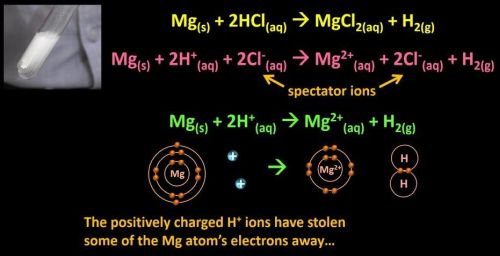Since the Cl ions appear on both sides and are only really spectator ions, we can also write Mg(s) + 2H+(aq) —> Mg2+(aq) + H2(g). Basically the positively charged H+ ions have stolen some of the Mg atom’s electrons away and have turned in hydrogen atoms while leaving the Mg atoms with an overall positive charge.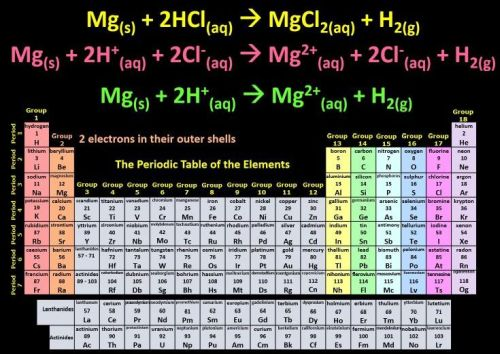Now, all atoms in Group 2 of the Periodic Table have 2 electrons in their outer shells and so they all lose two electrons when they react with acids. So these chemical equations would be exactly the same for all of them.  Group 1 elements have only one electron in their outer shells, so they only lose 1 electron when they react with acids.

So whenever any metal reacts with an acid (eg. Mg, Zn, Fe, Al), the H+ ions attract the outer electrons away from the metal atoms with more force than the metal atoms themselves are pulling on those electrons. In fact, many chemical reactions actually involve the forceful removal of electrons from one atom by another.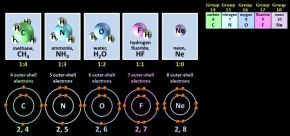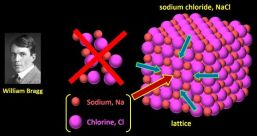For more on electrons shells, covalent bonding, and on the formation of ions, be sure to check out Episodes 6, 7, and 8 of the Shedding Light on Atoms series if you haven’t already.

Ultimately, all chemical reactions involve forces of attraction between the protons and electrons within and among atoms, and the strength of those forces determines whether two substances will chemically react. Acids are particularly reactive because the H+ ions they produce have a strong attraction to electrons. So, it’s bye for now. See you next time.

Credits:

Some simulations were produced by PhET Interactive Simulations, University of Colorado Boulder